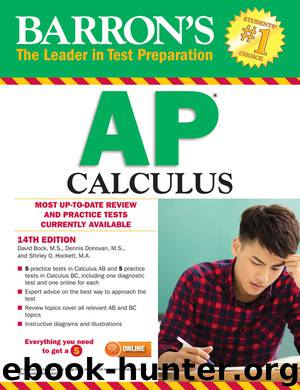﻿

# Barron's AP Calculus by David BockAuthor:David Bock
Language: eng
Format: epub
Publisher: Barron's Educational Series
Published: 2017-01-23T05:00:00+00:00

Figure N9–3a

Figure N9–3b

Figure N9–3c

Figure N9–3d

SOLUTIONS:

(A) goes with Figure N9–3c. The solution curves in the family y = sin x + C are quite obvious.

(B) goes with Figure N9–3a. The general solution is the family of parabolas y = x2 + C.

For (C) the slope field is shown in Figure N9–3b. The general solution is the family of cubics y = x3 − 3x + C.

(D) goes with Figure N9–3d; the general solution is the family of lines .

* * *

Example 4 __________________

(a)

Verify that relations of the form x2 + y2 = r2 are solutions of the d.e. .

(b)

Using the slope field in Figure N9–4 and your answer to (a), find the particular solution to the d.e. given in (a) that contains point (4,−3).